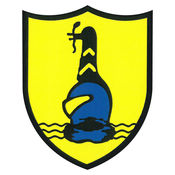Maths Questionnaire
Boughton Leigh Junior SchoolDo you like maths
Maths is my favourite subject
I am good at maths
I find maths easy
I am confident at maths
Maths is just about remembering facts
Getting the right answer is the most important thing in maths
Understanding how I am doing the maths is more important than the right answer.
I prefer working on my own in maths
I prefer to work with a partner in maths
When I work with a partner I prefer it to be a
Which part of maths do you find easy?
Which parts of maths do you find difficult?
If I am given a problem to solve, I have difficulty knowing where to start
Talking over maths problems with a friend or group helps with problem solving.
I have trouble getting started on a problem that is new to me
I like solving maths problems
I find drawing or using maths equipment helpful when solving maths problems